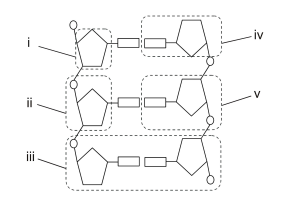# IMAT 2012 Q53 [Nucleotides]

Which one of the following labels, i to v, represents a nucleotide?A. i
B. iv
C. v
D. ii
E. iii

The answer to this question is C, where the figure labelled “v” is the nucleotide.

To begin answering this question, you must first be aware that this is a diagram of the DNA structure. It would be useful therefore, to be oriented to what the different shapes mean:

• Pentagon = the pentose sugar
• Circle = phosphate group
• Rectangle = nitrogenous base (purines and pyrimidines)

Next, you will need to be familiar with the difference between nucleo-S-ide and nucleo-T-ide:

• Nucleo-S-ide: This consists of the pentose sugar + nitrogenous base
• Nucleo-T-ide: This consists of the pentose sugar + nitrogenous base + phosphate group

Now that you are oriented to what the shapes mean and the different terms involved, let’s interpret the different labels:

• i: Pentose sugar only
• ii: Pentose sugar + phosphate group
• iii: Base pairing between 2 nucleotides
• iv: Pentose sugar + nitrogenous base = nucleoSide
• v: Pentose sugar + nitrogenous base + phosphate group = nucleoTide

Thus, the correct answer in this case will be choice C.